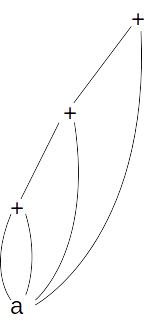##### Compiler-2M-Consider the following directed acyclic graph (DAG)

Consider the following directed acyclic graph (DAG):The expression represented by above DAG is:

(A) a + a+ (a + a + a)
(B) a + a+ (a + a + a +(a + a + a + a))
(C) a + a+ (a + a + a +(a + a + a ))
(D) None

A

B

C

D